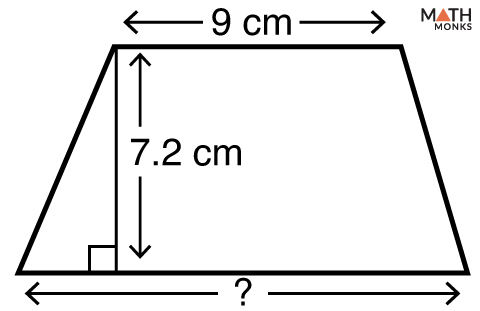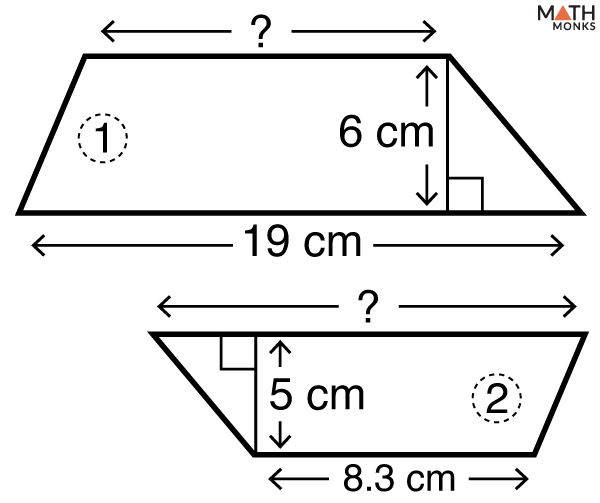# Base of a Trapezoid

A trapezoid has four sides – two parallel sides and two non-parallel sides. The parallel sides are its bases.

## Base Angles of a Trapezoid

An isosceles trapezoid has its two legs (non-parallel sides) congruent. So an isosceles trapezoid is a trapezoid with congruent base angles and congruent legs.

## Formula

The basic formula to find base of trapezoid given the perimeter is:

Now, let us solve an example to understand how to find the missing base of a trapezoid.

Find the base of a trapezoid whose perimeter is 45 cm, the long base is 15 cm, and the legs are 10 cm and 8 cm respectively.

Solution:

As we know,
a = P – b – c – d, here P = 45 cm, a = 15 cm, c = 10, d = 8 cm
∴a = 45 – 15 – 10 – 8
= 12 cm

Finding the missing base of a trapezoid when AREA, BASE and HEIGHT are knownFind the base of a trapezoid in the given figure whose area is 82.80 cm2.

Solution:

As we know,
Area (A) = ½ (a + b) × h, here A = 82.80 cm2, b = 9 cm, h = 7.2 cm, and a is unknown base
Now, solving for a,
a = 2A/h – b
= (2 × 82.80/7.2) – 9
= 14 cmFind the length of the base indicated for each trapezoid with a ‘?’, given in the figures, numbered 1 and 2. Given area of trapezoid 1 is 93 cm2 and trapezoid 2 is 53.25 cm2.

Solution:

Finding the missing base for trapezoid 1:
As we know,
b = 2A/h – a, here A = 93 cm2, h = 6 cm, a = 19 cm
= (2 × 93/6) – 9
= 22 cm
For trapezoid 2:
As we know,
a = 2A/h – b, here A = 53.25 cm2, h = 5 cm, b = 8.3 cm
= (2 × 53.25/5) – 8.3
= 13 cm